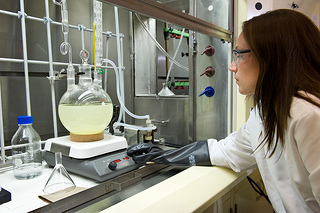## Ion Separation by Fractional Precipitation

#### Learning Objective

• Calculate the amount of an assay that will be required to ensure precipitation.

#### Key Points

• Fractional precipitation tests for certain ions using color change, solid formation, and other visible changes.
• Chlorides, bromides, and iodides can be detected by adding a small amount of silver nitrate solution.
• Sulfates can be detected with barium chloride solution.
• Carbonates can be detected with a small amount of acid.

#### Terms

• precipitationA reaction that leads to the formation of a heavier solid in a lighter liquid; the precipitate so formed at the bottom of the container.
• distilled waterWater that has been purified by distillation.

## Fractional Precipitation

Chemists are often presented with a sample and are asked to figure out what chemicals are in the compound or solution. One of the methods that can be applied to the solution is fractional precipitation. Fractional precipitation takes advantage of the different solubilities of ions to determine which ions are present in a solution. Testing for certain ions is accomplished using color change, solid formation, and other visible changes.Chemist analyzing unknown sampleU.S. Army scientists analyze unknown samples to determine whether hazardous chemical or biological warfare agents are present. Samples come from around the world.

## Example 1: Chloride or Carbonate Identification

Prepare a solution of the unknown salt using distilled water and add a small amount of silver nitrate (AgNO3) solution. If a white precipitate forms, the salt is either a chloride or a carbonate. Why?

If there is chlorine in the solution, the balanced reaction would be:

$Cl^{-} + Ag^{+} + NO_3^{-} \rightarrow AgCl + NO_3^{-}$

Silver chloride (AgCl) is not soluble and forms a white precipitate.

If there is carbonate in the solution, the balanced equation for the reaction would be:

${CO_{3}}^{2-} +2Ag^+ +2NO_3^{-} \rightarrow Ag_2CO_3 + 2NO_3^-$

Silver carbonate (Ag2CO3) is also not soluble in water.

So how does one tell if the precipitate is a carbonate or a chloride? Treat the precipitate with a small amount of concentrated nitric acid (HNO3). If the precipitate remains unchanged, then the salt is a chloride. If carbon dioxide is formed and the precipitate disappears, the salt is a carbonate. Here are the balanced equations:

$AgCl (s) + HNO_3 (l) \rightarrow$ (no reaction; precipitate is unchanged)

$Ag_2CO_3 (s) + 2HNO_3 (l) \rightarrow 2Ag^+ (aq) + 2NO_3^{-} (aq) + H_2O (l) + CO_2 (g)$

In the above reaction, the silver carbonate is used up and the precipitate will disappear.

## Example 2: Bromide or Iodide Identification

Bromides and iodides also form precipitates when they are reacted with silver nitrate, but both precipitates are pale yellow. To determine whether the precipitate is a bromide or iodide, use water with chlorine dissolved in it and the organic solvent carbon tetrachloride (CCl4). Chlorine water frees the bromine and iodine atoms as a gas. The bromine gas turns the CCl4 a reddish brown:

$2Br^{-} + Cl_2 \rightarrow 2Cl^{-} + Br_2$

$Br_2 + CCl_4 \rightarrow$ (reddish brown colored solution)

The iodine gas turns the CCl4 purple:

$2I^{-} + Cl_2 \rightarrow 2Cl^{-} + I_2$

$I_2 + CCl_4 \rightarrow$ (purple colored solution)

## Using Ksp in Fractional Precipitation

Solubility products (Ksp) can be used to complement the above methods. Knowing about the solubility of the ions can help determine how much of the assay compound needs to be added to guarantee precipitation.

For example, consider a solution the has 0.01 M barium chloride (BaCl2) and 0.02 M strontium chloride (SrCl2). Can either Ba2+ or Sr2+ be precipitated selectively with concentrated sodium sulfate (Na2SO4) solution? Which ion will precipitate first?

To find the solution, consider that the barium sulfate (BaSO4) solubility is given by:

$K_{sp} = [Ba^{2+}][{SO_{4}}^{2-}] = 1.5 \times 10^{-9}$

Rearrange the equation to solve for the concentration of SO42-. This will give you the value that the sulfate ion concentration must reach to precipitate BaSO4.

$[SO_4\ ^{2-}] = \frac{K_{sp}}{[Ba^{2+}]}$

With 0.01 M of Ba2+, barium sulfate will not precipitate until the sulfate ion concentration increases to:

$[SO_4\ ^{2-}] = \frac{1.5 \times 10^{-9}}{0.01}$

[SO42-] = 1.5 x 10-7

The Ksp for strontium sulfate (SrSO4) is 7.6 x 10-7.Strontium sulfate will precipitate when the sulfate concentration is:

$K_{sp} = [Sr^{2+}][{SO_{4}}^{2-}] = 7.6 \times 10^{-7}$

$[SO_4\ ^{2-}] = \frac{7.6 \times10^{-7}}{0.02}$

[SO42-] = 3.8 x 10-5

If the sulfate ion is slowly added to the container containing both the Ba2+ and Sr2+ ions, the barium sulfate will precipitate first. This is because it requires a lower concentration of the sulfate ion (1.5 x 10-7 < 3.8 x 10-5) to precipitate.